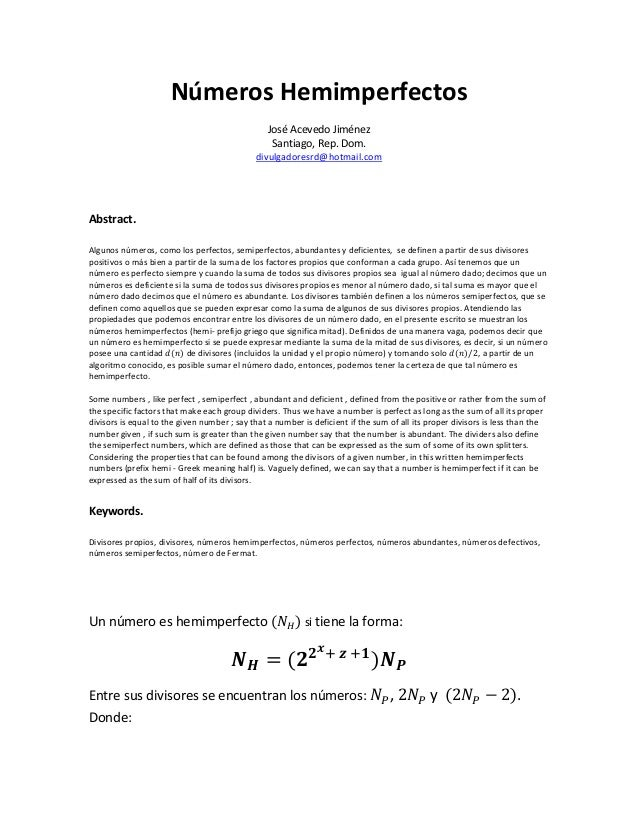Successfully reported this slideshow.Upcoming SlideShare
×

# Numeros hemimperfectos

414 views

Published on

Some numbers , like perfect , semiperfect , abundant and deficient , defined from the positive or rather from the sum of the specific factors that make each group dividers. Thus we have a number is perfect as long as the sum of all its proper divisors is equal to the given number ; say that a number is deficient if the sum of all its proper divisors is less than the number given , if such sum is greater than the given number say that the number is abundant. The dividers also define the semiperfect numbers, which are defined as those that can be expressed as the sum of some of its own splitters. Considering the properties that can be found among the divisors of a given number, in this written hemimperfects numbers (prefix hemi - Greek meaning half) is. Vaguely defined, we can say that a number is hemimperfect if it can be expressed as the sum of half of its divisors.

Published in: Education
• Full Name
Comment goes here.

Are you sure you want to Yes NoAre you sure you want to  Yes  No

Are you sure you want to  Yes  No

Are you sure you want to  Yes  No

Are you sure you want to  Yes  No